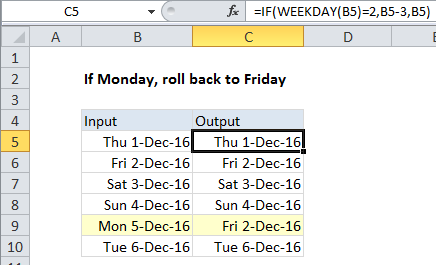## Excel Office

Excel How Tos, Tutorials, Tips & Tricks, Shortcuts

# Roll back weekday to Friday base on a particular date in Excel

## Case study:

If Monday, roll back to Friday

To check the weekday of a date, and roll back to Friday when the date is a Monday, you can use the IF and WEEKDAY functions.

## Formula

`=IF(WEEKDAY(date)=2,date-3,date)`## Explanation

In the example shown, the formula in C5 is

`=IF(WEEKDAY(B5)=2,B5-3,B5)`

### How this formula works

The WEEKDAY function returns a number, 1-7, that corresponds to particular days of the week. By default, WEEKDAY assumes a Sunday-based week, and assigns 1 to Sunday, 2 to Monday, and so on, with 7 assigned to Saturday.

Worked Example:   If this AND that OR that in Excel

In this case, we only want to take action if the date in question is Monday. To test, we use this expression inside the IF function:

`WEEKDAY(B5)=2`

If the logical expression returns TRUE, we know the date is a Monday, so we subtract 3 to “roll back” to Friday. If the expression returns FALSE, we simply return the original date.

Worked Example:   Calculate Conditional Percentile 'IF' in table in Excel